# Among the following compounds,

Question:

Among the following compounds, which one has the shortest $\mathrm{C}-\mathrm{Cl}$ bond ?

1.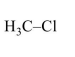2.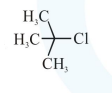3.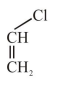4.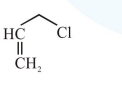Correct Option: , 3

Solution: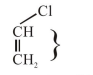In option (3) $\mathrm{C}-\mathrm{Cl}$ bond is shortest due to resonance of lone pair of $-\mathrm{Cl}$.

Due to resonance $\mathrm{C}-\mathrm{Cl}$ bond acquire partial double bond character.

Hence $\mathrm{C}-\mathrm{Cl}$ bond length is least.